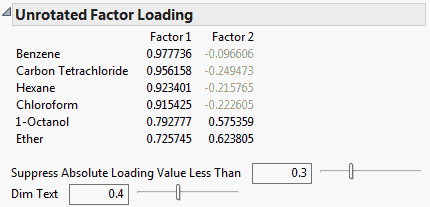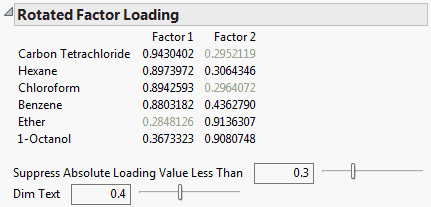Multivariate Methods > Factor Analysis > Factor Analysis Model Fit Options
Publication date: 05/24/2021

# Factor Analysis Model Fit Options

The Factor Analysis Model Fit red triangle menu contains the following options:

Prior Communality

(Available only for Common Factor Analysis.) Shows or hides an initial estimate of the communality for each variable. For a given variable, this estimate is the squared multiple correlation coefficient (SMC), or RSquare, for a regression of that variable on all other variables.

Eigenvalues

(Available only for Common Factor Analysis.) Shows or hides the eigenvalues of the reduced correlation matrix and the percent of the common variance for which they account. The reduced correlation matrix is the correlation matrix with its diagonal entries replaced by the communality estimates. The eigenvalues indicate the common variance explained by the factors. The Cum Percent can exceed 100% because the reduced correlation matrix is not necessarily positive definite and can have negative eigenvalues.

Note the table indicates the number of factors retained for analysis.

Shows or hides the factor loading matrix before rotation. Factor loadings measure the influence of a common factor on a variable. Because the unrotated factors are orthogonal, the factor loading matrix is the matrix of correlations between the variables and the factors. The closer the absolute value of a loading is to 1, the stronger the effect of the factor on the variable.

Use the slider and value to Suppress Absolute Loading Values Less Than the specified value in the table. Suppressed values appear dimmed according to the setting specified by Dim Text.

Use the Dim Text slider and value to control the table’s font transparency gradient for factor values less in absolute value than the specified Suppress Absolute Loading Values Less Than value.Note: The Unrotated Factor Loading matrix is ordered so that variables associated with the same factor appear next to each other.

Rotation Matrix

Interfactor Correlations

(Available only for oblique rotations.) Shows or hides the matrix of correlations between factors.

Target Matrix

(Available only for the Promax rotation.) Shows or hides the matrix to which the varimax factor pattern is rotated.

Factor Structure

(Available only for oblique rotations.) Shows or hides the matrix of correlations between variables and common factors.

Final Communality Estimates

Shows or hides estimates of the communalities after the factor model has been fit. When the factors are orthogonal, the final communality estimate for a variable equals the sum of the squared loadings for that variable.

Standard Score Coefficients

Shows or hides a table of the multipliers used to estimate factor scores when saving rotated factors to the source data table.

Variance Explained by Each Factor

(Available only for orthogonal rotations.) Shows or hides the variance, percent, and cumulative percent, of common variance explained by each rotated factor.

Variance Explained by Each Factor Ignoring Other Factors

(Available only for oblique rotations.) Shows or hides the variance and percent of common variance explained by each rotated factor regardless of other factors.

Significance Test

(Available only for the Maximum Likelihood factoring method.) Provides the results of two Chi-square tests.

The first test is for H0: No common factors. This null hypothesis indicates that none of the common factors explain the intercorrelations among the variables. This test is Bartlett’s Test for Sphericity, whose null hypothesis is that the correlation matrix of the factors is an identity matrix (Bartlett, 1954).

The second test is for H0: N factors are sufficient, where N is the specified number of factors. Rejection of this null hypothesis indicates that more factors might be required to explain the intercorrelations among the variables (Bartlett, 1954). The Criterion is the log-likelihood objective function value.

Measures of Fit

(Available only for the Maximum Likelihood factoring method.) Shows or hides measures of fit: Chi-Squared without Bartlett’s Correction, AIC, BIC, Tucker-Lewis’s Index, and the root mean square error of approximation.

Measures of Factor Scores

Shows or hides measures of factor score determinacy: Multiple R, Multiple R Square, and Minimum Correlation. These measures are used to evaluate if the factor scores might be useful for secondary analyses.

Shows or hides the factor loading matrix after rotation. If the rotation is orthogonal, these values are the correlations between the variables and the rotated factors.

Use the slider and value to Suppress Absolute Loading Values Less Than the specified value in the table. Suppressed values appear dimmed according to the setting specified by Dim Text.

Use the Dim Text slider and value to control the table’s font transparency gradient. The lower the value, the more transparency the font for factor values less in absolute value than the specified Suppress Absolute Loading Values Less Than value.Note: The Rotated Factor Loading matrix is ordered so that variables associated with the same factor appear next to each other.

Shows or hides a plot of the rotated factor loadings. When more than 2 factors are modeled, the loading plot is a matrix of plots.

Score Plot

Shows or hides a scatterplot of the estimated factor scores. When more than 2 factors are modeled, the score plot is a matrix of plots.

Score Plot with Imputation

(Available only if there are missing values.) Shows or hides a scatterplot of the estimated factor scores with imputed values for missing values.

Display Options